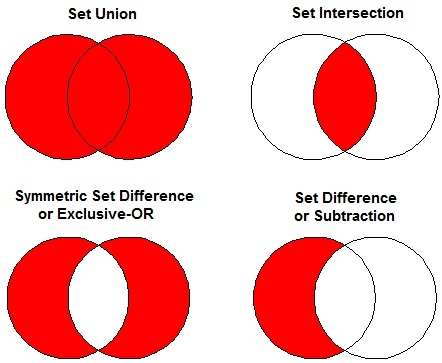# C++ Program to Implement Set_Symmetric_difference in STL

This is a c++ program to implemet set_symmetric_difference. The symmetric difference of two sets is built by the elements that are present in one of the sets, but not in the other.

Common set operations are −

• Set Union
• Set Intersection
• Symmetric Set Difference or Exclusive-OR
• Set Difference or Subtraction## Algorithm

Begin
Declare set vector v and iterator st.
Initialize st = set_symmetric_difference (set1, set1 + n, set2, set2 +n, v.begin()))
Print the elements obtained after symmetric_difference of two sets
End.

## Example Code

#include<iostream>
#include <algorithm>
#include <vector>
using namespace std;
int main () {
int set1[] = {5,6,7,8,9,10};
int set2[] = {1,2,3,4,6,7};
vector<int> v(10);
vector<int>::iterator st;
sort (set1, set1 + 6);
sort (set2, set2 + 6);
st = set_symmetric_difference(set1, set1 + 6, set2, set2 + 6, v.begin());
v.resize(st - v.begin());
cout<<"The symmetric difference between the sets has "<< (v.size())<< " elements: "<<endl;
for (st = v.begin(); st != v.end(); ++st)
cout<< *st<<" ";
cout <<endl;
return 0;
}

## Output

The symmetric difference between the sets has 8 elements:
1 2 3 4 5 8 9 10